# GATE Past Year Questions: Radiation - Heat Transfer - Mechanical Engineering

Question for GATE Past Year Questions: Radiation
Try yourself:For a glass plate transmissivity and reflectivity are specified as 0.86 and 0.08 respectively, the absorptivity of the glass plate is



Question for GATE Past Year Questions: Radiation
Try yourself:A diffuse radiation surface has



Question for GATE Past Year Questions: Radiation
Try yourself:What is the value of the view factor for two inclined flat plates having common edge of equal length and wlth an angle of 20 degrees?



Question for GATE Past Year Questions: Radiation
Try yourself:The following figure was generated from experimental data relating spectral black body emissive power to wave length at the three temperatures T1, T2 and T3( T1 > T2 >T3).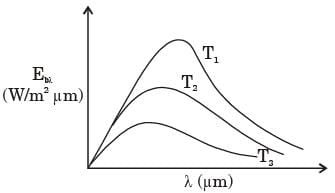The conclusion in that the measurements are



Question for GATE Past Year Questions: Radiation
Try yourself:For an opaque surface, the absorptivity (α), transmissivity (τ) and reflectivity (ρ) are related by the equation



Question for GATE Past Year Questions: Radiation
Try yourself:The radiative heat transfer rate per unit area (W/m2) between two plane parallel gray surfaces (emissivity = 0.9) maintained at 400 K and 300 K is (σ = Stefan Boltzmann constant = 5.67 × 10-8 W/m2K4)



Question for GATE Past Year Questions: Radiation
Try yourself:A plate having 10 cm2 area each side is hanging in the middle of a room of 100 m2 total surface area. The plate temperature and emissivity are respectively 800 K and 0.6. The temperature and emissivity valute for the surfaces of the room are 300 K and 0.3 respectively. Boltzmann's constant σ = 5.67 × 10-8 W/m2K4. The total heat loss from the two surfaces of the plate is



Question for GATE Past Year Questions: Radiation
Try yourself:A100 W electric bulb was switched on in a 2.5 m × 3 m × 3 m size thermally insulated room having a temperature of 20°C. The room temperature at the end of 24 hours will be



Question for GATE Past Year Questions: Radiation
Try yourself:Radiative heat transfer is intercepted between the inner surfaces of two very large isothermal parallel metal plates. While the upper plate (designated as plate 1) is a black surface and is the warmer one being maintained at 727°C, the lower plate (plate 2) is a diffuse and gray surface with an emissivity of 0.7 and is kept at 227°C. Assume that the surfaces are sufficiently large to form a two-surface enclosure and steady-state conditions to exist. Stefan-Boltzmann constant is given as 5.67 × 10–8 W/m2K4.

The irradiation (in kW/m2) for the plate (plate 1) is



Question for GATE Past Year Questions: Radiation
Try yourself:Radiative heat transfer is intercepted between the inner surfaces of two very large isothermal parallel metal plates. While the upper plate (designated as plate 1) is a black surface and is the warmer one being maintained at 727°C, the lower plate (plate 2) is a diffuse and gray surface with an emissivity of 0.7 and is kept at 227°C. Assume that the surfaces are sufficiently large to form a two-surface enclosure and steady-state conditions to exist. Stefan-Boltzmann constant is given as 5.67 × 10–8 W/m2K4.

If plate 1 is also a diffuse and gray surface with an emissivity value of 0.8, the net radiatibn heat exchange (in kW/m2) between plate 1 and plate 2 is



Question for GATE Past Year Questions: Radiation
Try yourself:Two larged if fuse gray parallel plates, separated by a small distance, have surface temperature of 400 K and 300 K. If the emissivities of the surfaces are 0.8 and the Stefan-Boltzmann constant is 5.67 × 10–8 W/ m2K4, the net radiation heat exchange rate in kW/m2 between the two plates is



Question for GATE Past Year Questions: Radiation
Try yourself:The total emissive power of a surface is 500 W/ m2 at temperature T1 and 1200 W/m2 at emperature T2, where the temperature are in Kelvin. Assuming the emissivity of the surface to be constant, the ratio of the temperature T1/T2 is



Question for GATE Past Year Questions: Radiation
Try yourself:The emissive power of a blackbody is P. If its absolute temperature is doubled, the emissive power becomes



Question for GATE Past Year Questions: Radiation
Try yourself:The peak wavelength of radiation emitted by a black body at a temperature of 2000 K is 1.45 μm. If the peak wavelength of emitted radiation changes to 2.90 μm , then the temperature (in K) of the black body is



Question for GATE Past Year Questions: Radiation
Try yourself:For the circular tube of equal length and diameter shown in figure below, the view factor F13 is 0.17. The view factor F12 in this case will be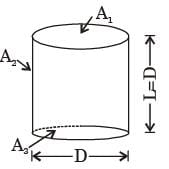Question for GATE Past Year Questions: Radiation
Try yourself:A solid cylinder (surface 2) is located at the centre of a hollow sphere (surface 1). The diameter of the sphere is 1 m, while the cylinder has a diameter and length of 0.5 m each.'The radiation configuration factor F11 is



Question for GATE Past Year Questions: Radiation
Try yourself:A hollow enclosure is formed between two inf initely long concentric cylinders of radii 1 m and 2 m, respectively. Radiative heat exchange takes place between the inner surface of the larger cylinder (surface-2) and the outer surface of the smaller cylinder (surface-1). The radiating surface are diffuse and the medium in the enclosure is non- participating. The fraction of the thermal radiation leaving the larger surface and striking itself is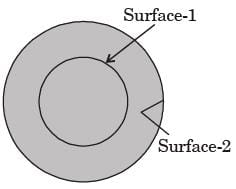Question for GATE Past Year Questions: Radiation
Try yourself:Consider two infinitely long thin concentric tubes of circular cross section as shown in figure. If D1 and D2 are the diameters of the inner and outer tubes respectively, then the view factor F22 is given by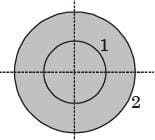Question for GATE Past Year Questions: Radiation
Try yourself:A solid sphere of radius r1 = 20 mm is placed concentrically inside a hollow sphere of radius r2 = 30 mm as shown in the figure.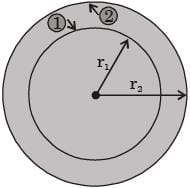The view factor F21 for radiation heat transfer is



Question for GATE Past Year Questions: Radiation
Try yourself:A solid sphere 1 of radius r is placed inside a hollow, closed hemispherical surface 2 of radius 4r. The shape factor F is,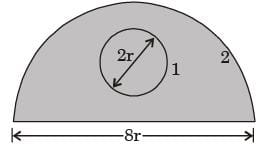Question for GATE Past Year Questions: Radiation
Try yourself:Consider the radiation heat exchange inside an annulus between two very long concentric cylinders. The radius of the outer cylinder is R0 and that of the inner cylinder is Ri. The radiation view factor of the outer cylinder onto itself is



Question for GATE Past Year Questions: Radiation
Try yourself:Sphere 1 with a diameter of 0.1 m is completely enclosed by another sphere 2 of diameter 0.4 m.The view factor F12 is



Question for GATE Past Year Questions: Radiation
Try yourself:Two infinite parallel plates are placed at a certain distance apart. An infinite radiation shield is inserted between the plates without touching any of them to reduce heat exchange between the plates. Assume that the emissivities of plates and radiation shield are equal. The ratio of the net heat exchange between the plates with and without the shield is



The document GATE Past Year Questions: Radiation | Heat Transfer - Mechanical Engineering is a part of the Mechanical Engineering Course Heat Transfer.
All you need of Mechanical Engineering at this link: Mechanical Engineering

## Heat Transfer

58 videos|69 docs|86 tests

## FAQs on GATE Past Year Questions: Radiation - Heat Transfer - Mechanical Engineering

 1. What is radiation?Ans. Radiation is the emission of energy as electromagnetic waves or as moving subatomic particles, especially high-energy particles that cause ionization.
 2. What are the different types of radiation?Ans. There are several types of radiation, including electromagnetic radiation (such as X-rays and gamma rays) and particulate radiation (such as alpha particles and beta particles).
 3. How does radiation affect the human body?Ans. Radiation can have harmful effects on the human body, depending on the dose and type of radiation. It can damage cells, DNA, and other biological molecules, leading to health issues such as cancer, radiation sickness, and genetic mutations.
 4. What are the sources of radiation exposure?Ans. Radiation exposure can come from various sources, including natural sources such as cosmic rays, radioactive elements in the earth's crust, and radon gas, as well as man-made sources such as medical imaging procedures, nuclear power plants, and industrial activities involving radioactive materials.
 5. How can one protect themselves from radiation exposure?Ans. There are several ways to protect oneself from radiation exposure. These include minimizing unnecessary exposure, using protective shielding or clothing, following safety protocols in radioactive environments, and ensuring proper monitoring and containment measures in industries dealing with radioactive materials.

## Heat Transfer

58 videos|69 docs|86 testsExplore Courses for Mechanical Engineering examSignup to see your scores go up within 7 days! Learn & Practice with 1000+ FREE Notes, Videos & Tests.
10M+ students study on EduRev
Track your progress, build streaks, highlight & save important lessons and more!
Related Searches

,

,

,

,

,

,

,

,

,

,

,

,

,

,

,

,

,

,

,

,

,

;